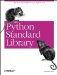# The timing Module

(Obsolete, Unix only) The timing module can be used to time the execution of a Python program. Example 14-25 demonstrates.

##### Example 14-25. Using the timing Module
```File: timing-example-1.py

import timing
import time

def procedure():
time.sleep(1.234)

timing.start()
procedure()
timing.finish()

print "seconds:", timing.seconds()
print "milliseconds:", timing.milli()
print "microseconds:", timing.micro()

seconds: 1
milliseconds: 1239
microseconds: 1239999```

The script in Example 14-26 shows how you can emulate this module using functions in the standard time module.

##### Example 14-26. Emulating the timing Module
```File: timing-example-2.py

import time

t0 = t1 = 0

def start():
global t0
t0 = time.time()

def finish():
global t1
t1 = time.time()

def seconds():
return int(t1 - t0)

def milli():
return int((t1 - t0) * 1000)

def micro():
return int((t1 - t0) * 1000000)```

You can use time.clock() instead of time.time() to get CPU time, where supported.Python Standard Library (Nutshell Handbooks) with
ISBN: 0596000960
EAN: 2147483647
Year: 2000
Pages: 252
Authors: Fredrik Lundh